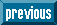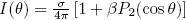## Indicators

•Cited by SciELO
•Access statistics

•Similars in SciELO

## Print version ISSN 0035-001X

### Rev. mex. fis. vol.58 n.2 México Apr. 2012

Erratum

L.H. Haber, B. Doughty, and S.R. Leone: "Photoelectron velocity map imaging of single–photon and pump–probe ionization of noble gases using femtosecond high–order harmonics," Rev. Mex. Fis. S 56(2) (2010) 85–87.

• The complete 3rd paragraph of page 85 should read:

"The photoelectron angular distributions of randomly oriented atoms and molecules from single–photon ionization using linearly polarized light is given bywhere a is the total cross section, θ is the angle between the laser polarization and electron velocity vectors, and β is the anisotropy parameter associated with the second order Legendre polynomial . For atoms, the anisotropy parameter can be described using the equation,where Δ = δl+1 – δl–1 . Here, δl±1 is the phase shift and Dl±1is the radial dipole matrix element of the partial wave of angular momentum l ± 1. For an electron originally in an atomic orbital of angular momentum l, the outgoing partial waves will have angular momenta of l ± 1 and the interference pattern of these partial waves results in the observed photoelectron angular distributions. The radial dipole matrix elements and the phase shift differences depend on photoelectron energy and provide information on both the bound and continuum wavefunctions. The anisotropy parameters, therefore, provide detailed information on properties of photoionization."

• The first complete paragraph of page 86 should read:

"Inverted images from single–photon ionizations of He, Ne, Ar, and Xe are shown in Figs. 1a–1d. The anisotropy parameter, β, is evaluated at the peak radius for each ring. Using the 17th harmonic (26.5 eV), the β values of He and Ne are 2.03 ± 0.05 and 0.24 ± 0.01, respectively. Using the 13th harmonic (20.2 eV), the β values for the ionization of Ar to the 2P3/2 state and Xe to the 2P1/2 and 2P3/2 states are 0.85 ± 0.06, 1.59 ± 0.07, and 1.72 ± 0.04, respectively. For He, there is only one partial wave, the P wave, and the expected β value of 2.00 is in agreement with the measurements. Additional photoelectron images from single photon ionization of Ne, Ar, and Xe were taken with the 11th and 13th harmonics. Using the 11th harmonic (17.1 eV) the β values for the ionizations of Ar to the 2P3/2 state and Xe to the 2P1/2 and 2P3/2states are 0.27 ± 0.05, 1.05 ± 0.10, and 1.45 ± 0.01, respectively. Using the 15th harmonic (23.4 eV), the β values for the ionization of Ne and Ar are –0.16 ± 0.03 and 1.27 ± 0.01, respectively. All reported anisotropy parameters are in excellent agreement with literature values [7–9]."All the contents of this journal, except where otherwise noted, is licensed under a Creative Commons Attribution License